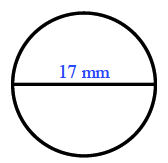### Home > CC2MN > Chapter 10 > Lesson 10.3.4 > Problem10-102

10-102.

Find the area and circumference of a circle that has a diameter of $17$ mm. Write your answers in terms of $\pi$ and as a decimal approximation.

Use the formulas for the area and circumference of a circle:

Area: $\pi\cdot r^2$
Circumference: $\pi·2r$ or $\pi·d$$\text{Area}=\pi(8.5)^2=72.25\pi\approx226.98\ \text{mm}^2$